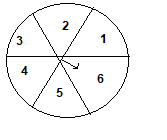# Extra Questions for Probability for Class 10 Maths

Given below are the Class 10 Maths Extra Questions for Probability
a. Concepts questions
b. Calculation problems
c. Multiple choice questions
e. Fill in the blank's
Question 1.
An unbiased die is thrown.
Find out the probabilities of getting
a. Prime Number
b. odd number
c. even number
d. 0
e. A multiple of 2 and 3
f. Number less than 7
g. A multiple of 4

Question 2.
In a charity show tickets numbered consecutively from 101 through 350 are placed in a box.
What is the probability that a ticket selected at random (blindly) will have a number with a hundredth digit of 2?

Question 3
If p is chosen at random from the set {1,2,3,4} and q is to be chosen at random from the set {5,6,7}, what is the probability that pq will be even?

Question 4
A dice is thrown and 2 comes, the dice is again thrown, what is the probability of getting score higher than first throw
(a) 20%
(b) 66.67%
(c) 25 %
(d)33%

Question 5
John and Tom are friends. What is the probability that both will have?
(i) the same birthday?
(ii) different birthdays?
(iii) their birthday on the same weekday?

Question 6
One card is drawn from a pack of 52 cards.
What is the probability of getting?
(i) an ace or a king?
(ii) a red card and a king?
(iii) a face card?
(iv) a king or a queen or a jack?

Question 7
A disc on which a player spins an arrow twice. The disc has numbers from 1 -6 . The fraction  a/b is formed where 'a' is the number of sector on which the arrow stops on first spin and 'b' is the number of sector in which the arrow stops on the second spin. on each spin , each sector has equal chance of selection by the arrow. Find the probability that the fraction a/b > 1Question 8
A bag contains white,black and red balls only. A ball is drawn at random from the bag. If the probability of getting white ball is 3/10 and that of black ball os 2/5.
i. Find the probability of drawing red ball
ii. If the bag contains 20 black balls,find the total number of balls in the bag

Question 9
If p is chosen at random from the set {1,2,3,4} and q is to be chosen at random from the set {1,4,9,16}, what is the probability that pq will be less than 16?

Question 10
A piggy bank contains hundred 50 p coins,seventy Rs 1 coin, fifty Rs 2 coin and thirty Rs 5 coins.It is equally likely that one of of the coin will fall out when the bank ios turned upside down.What is the probability  that the coin
a. will be Rs 1 coin
b. will not be Rs 5 coin
c.  will be 50 p coin or Rs 2 coin

2. 100/249
3.   7/12
4.  b
7. 5/12
8. 3/10, 50
9. 1/2
10. 7/25, 22/25,3/5Go back to Class 10 Main Page using below links

### Practice Question

Question 1 What is $1 - \sqrt {3}$ ?
A) Non terminating repeating
B) Non terminating non repeating
C) Terminating
D) None of the above
Question 2 The volume of the largest right circular cone that can be cut out from a cube of edge 4.2 cm is?
A) 19.4 cm3
B) 12 cm3
C) 78.6 cm3
D) 58.2 cm3
Question 3 The sum of the first three terms of an AP is 33. If the product of the first and the third term exceeds the second term by 29, the AP is ?
A) 2 ,21,11
B) 1,10,19
C) -1 ,8,17
D) 2 ,11,20